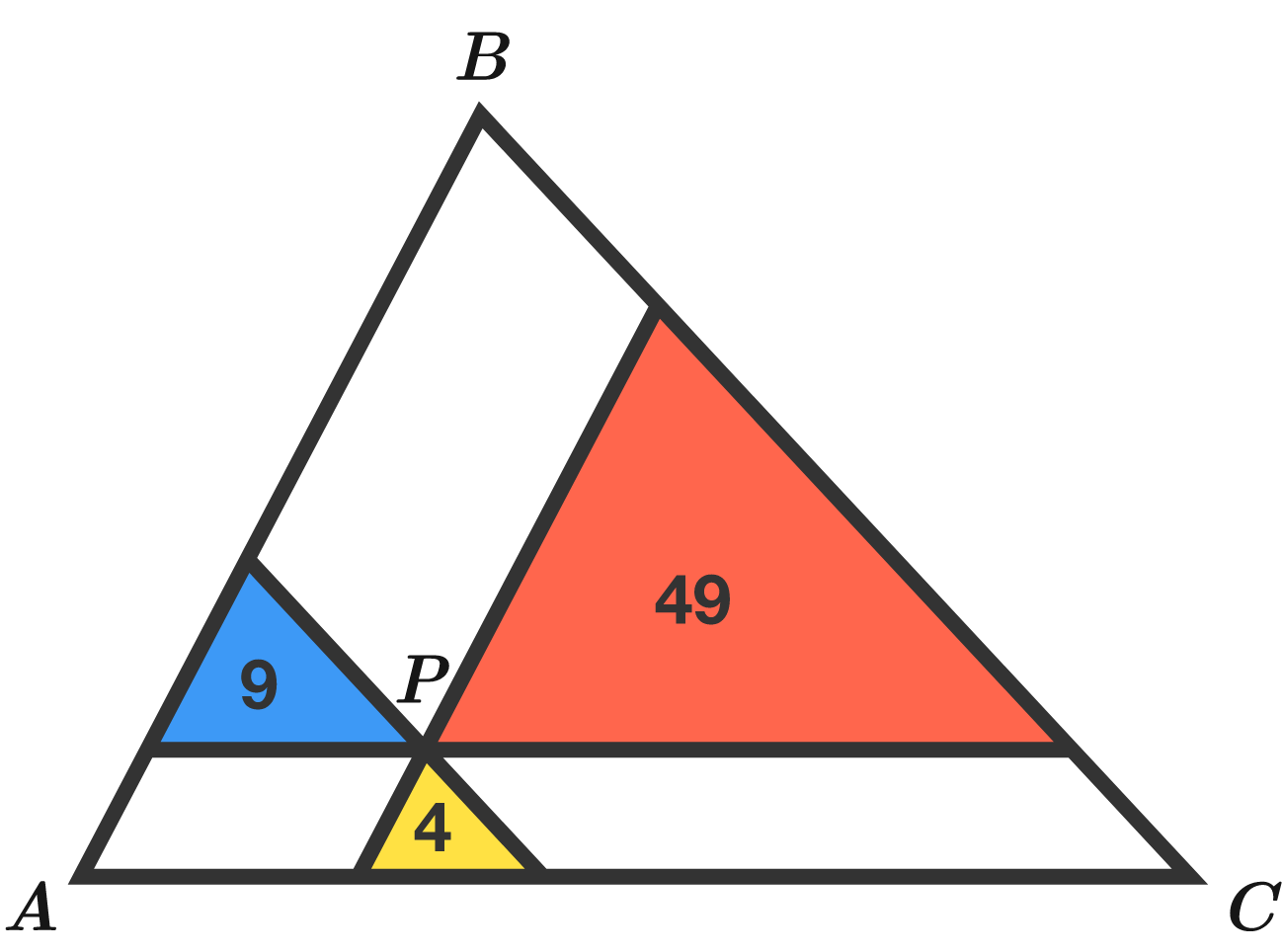# Straight lines coordinate geometry

Geometry Level 2

Lines are drawn through point $P$ parallel to the sides of triangle $ABC.$ The resulting 3 triangles have areas 4, 9, and 49.

What is the area of triangle $ABC?$The areas of these 3 smaller triangles are 4, 9, and 49. What's the area of the big, outer triangle?

×